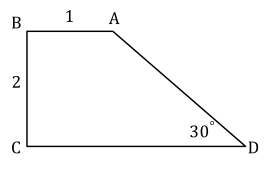# Free SAT Practice Questions

Question 1 of 1
ID: SAT-ATIM-3
Section: Math - Additional Topics in Math
Topics: Geometry; Quadrilateral
Difficulty level: Challenging

(Practice Mode: Single selected Question » Back to Overview)In the figure given above, what is the sum of the lengths of AD and CD to the nearest tenth? (With calculator)

Numeric Answer:

General Hint: Enter the answer as an integer or a decimal in a single answer box. If two answer boxes are given, enter the answer as a fraction, with one box being the numerator and the other being the denominator. Read carefully whether the answer should rounded and make sure you round to the required degree of accuracy. Do not enter any symbols or characters in the box(es) provided.